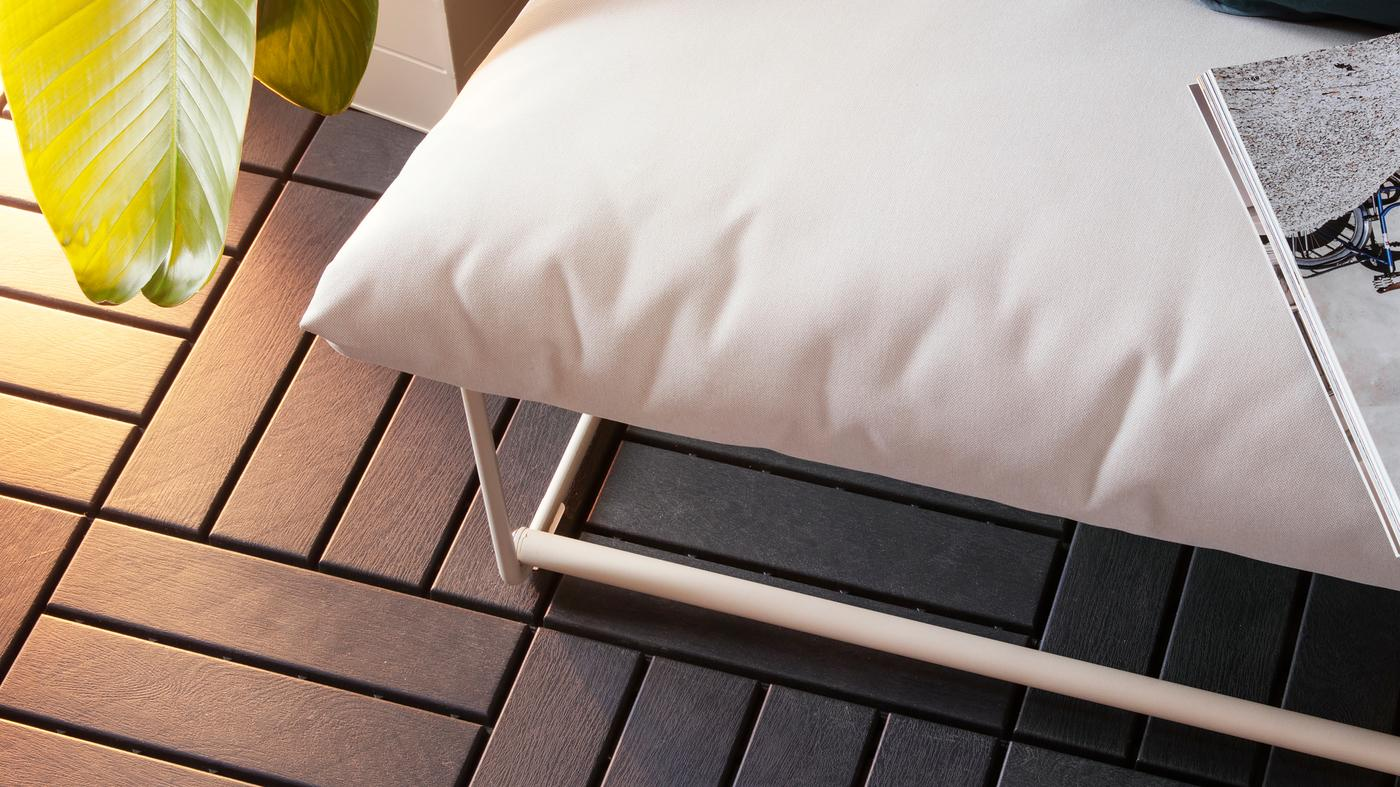Quickly create a personal look with a range of outdoor floor decking in different materials and colours. They click together, so it is easy to upgrade your outdoor flooring.## 1. Calculate the size of your space

To calculate your outdoor area, multiply the length by the width. For example, width 3 m × 5 m = 15 m2. Calculate any areas to be subtracted from the total area, like pillars or corners. For example, corner width 0.5 m × length 1.0 m = 0.5 m². The correct subtracted area is then: 15 m² – 0.5 m² = 14.5 m².

## 2. Calculate how many packages you need

Divide the sum by 0.81 and round up (to ensure enough floor decking). For example, 14.5 m2 ÷ 0.81 = 17.90. Rounded up, that means you need 18 packages.

When you have your total area of space, these calculations tell you how many flooring packages are needed to cover it.

The most common examples:

2 m2 needs 3 packages

5 m2 needs 7 packages

7 m2 needs 9 packages

10m2 needs 13 packages

12m2 needs 15 packages

18m2 needs 23 packages

20m2 needs 25 packages

25m2 needs 31 packages

30m2 needs 37 packages